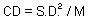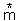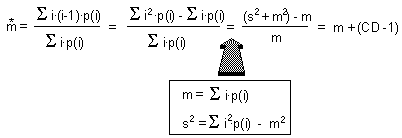## 3.4. Indexes of aggregation

1. Coefficient of dispersion:2. Mean crowding(Lloyd 1967) is equal to the mean number of "neighbors" in the same quad:
 Sample No. No. of individuals (N) No. of neighbors (N-1) N(N-1) 1 5 4 20 2 3 2 6 3 0 -1 0 4 1 0 0 5 7 6 42 Total 16 - 68

It means that the mean number of "neighbors" is= 4.25.

Note: mean crowding has biological sense only if the size of each quad corresponds to "interaction distance" among individuals.

Consider probability distribution p(i) = the proportion of samples with i individuals. Then:For a random (poisson) distribution, CD=1, and thus,= m.

3. Lloyd (1967) suggested a "patchiness" index:/m, which is 1 for a random distribution, > 1 for aggregated distributions, and <1 for regular distributions. However it does not make more sense than CD.

Alexei Sharov 12/28/95